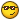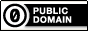# Conway's Life Game

## Recommended Posts

This is a small one, I guess everybody knows itApzo.

```Opt("GUIOnEventMode", 1)
#include <GUIConstants.au3>

Global Const \$version = "V. 0.99"
Global Const \$H_Sep = 22
Global \$MainG

Global \$Refresh = 20    ;sleeping time between 2 states, in ms
Global Const \$GridSize = 25;Toric grid, 25x25
Global Const \$T = 10    ;cell size, in pixels

Global \$G_Width = \$GridSize * \$T +2
Global \$G_Height = \$GridSize * \$T + 70

Global \$Grid[\$GridSize][\$GridSize]
Global \$cells
Global \$turns=0
Global \$GuiNbCells
Global \$GuiNbTurn = 0

\$MainG = GUICreate("Life Game " & \$version, \$G_Width, \$G_Height, -1, -1, \$WS_SIZEBOX+\$WS_MINIMIZEBOX+\$WS_EX_ACCEPTFILES)
GUISetOnEvent(\$GUI_EVENT_CLOSE, "CLOSEClicked")
GUISetFont(-1, -1, -1, "Courier New");monospaced font for better display
GUISetState()

;~ Generate grid controls and randomly fills it
For \$x=0 to \$GridSize-1
For \$y=0 to \$GridSize-1
If Random(1,3) > 2 Then
\$Grid[\$x][\$y] = GUICtrlCreateLabel("*", \$x*\$T, \$y*\$T, \$T, \$T)
\$Grid[\$x][\$y] = "*"
\$cells = \$cells +1
Else
\$Grid[\$x][\$y] = GUICtrlCreateLabel(" ", \$x*\$T, \$y*\$T, \$T, \$T)
\$Grid[\$x][\$y] = " "
EndIf
Next
Next

\$GuiNbCells = GUICtrlCreateLabel("Cells : " & \$cells & "   ", 2, \$GridSize * \$T + 2)
\$GuiNbTurns = GUICtrlCreateLabel("Turns : " & \$turns & "   ", 2, \$GridSize * \$T+ 14)

While 1
For \$x=0 to \$GridSize-1
For \$y=0 to \$GridSize-1
;~          checks neighbours
\$neigh=0
\$xm1=Mod(\$x-1+\$GridSize, \$GridSize)
\$xp1=Mod(\$x+1, \$GridSize)
\$ym1=Mod(\$y-1+\$GridSize, \$GridSize)
\$yp1=Mod(\$y+1, \$GridSize)
If \$Grid[\$xm1][\$ym1] = "*" Then
\$neigh = \$neigh + 1
EndIf
If \$Grid[\$xm1][\$y] = "*" Then
\$neigh = \$neigh + 1
EndIf
If \$Grid[\$xm1][\$yp1] = "*" Then
\$neigh = \$neigh + 1
EndIf
If \$Grid[\$x][\$ym1] = "*" Then
\$neigh = \$neigh + 1
EndIf
If \$Grid[\$x][\$yp1] = "*" Then
\$neigh = \$neigh + 1
EndIf
If \$Grid[\$xp1][\$ym1] = "*" Then
\$neigh = \$neigh + 1
EndIf
If \$Grid[\$xp1][\$y] = "*" Then
\$neigh = \$neigh + 1
EndIf
If \$Grid[\$xp1][\$yp1] = "*" Then
\$neigh = \$neigh + 1
EndIf
\$action = ""

;~          Actually I use 2 grids : one represents the n+0 state,
;~          the second: state at n+1

;~          Cell dies if less than 2 neighbours, or more than 3
\$Grid[\$x][\$y] = \$Grid[\$x][\$y]
If \$Grid[\$x][\$y] = "*" And (\$neigh < 2 Or \$neigh > 3) Then
;~              Cell death
\$Grid[\$x][\$y]=" "
\$cells = \$cells - 1
\$action = "Farewell, cruel world."
EndIf

;~          Cells comes to life if exactly 3 neighbours
If \$Grid[\$x][\$y] = " " And \$neigh = 3 Then
;~              Cell birth
\$Grid[\$x][\$y]="*"
\$cells = \$cells + 1
\$action = "Hello, world!."
EndIf
Next
Next

;~  Refreshing the current grid, n+1 becomes n+0
For \$x=0 to \$GridSize-1
For \$y=0 to \$GridSize-1
\$Grid[\$x][\$y] = \$Grid[\$x][\$y]
GuiCtrlSetData(\$Grid[\$x][\$y], \$Grid[\$x][\$y])
Next
Next
\$turns = \$turns + 1
GuiCtrlSetData(\$GuiNbCells, "Cells : " & \$cells)
GuiCtrlSetData(\$GuiNbTurns, "Turns : " & \$turns)
Sleep(\$Refresh)
Wend

Func CLOSEClicked()
Exit
EndFunc```
##### Share on other sites

Pretty cool work! GJ! Its fun to watch, but it stops at one point, something like 180 rounds.

I mean it doesn't stop but every cell has 2 neighbors and nothing happens anymore. I would add something that adds like 5 cells randomly every 5 rounds or something, that would make the script more random!

Clean coding! Keep that up

Felix N. (tdlrali)

##### Share on other sites
• 4 years later...

```C:\Program Files\AutoIt3\Examples\GameBot - Builder\Conway's Life Game.au3 (21) : ==> Variable used without being declared.:
\$MainG = GUICreate("Life Game " & \$version, \$G_Width, \$G_Height, -1, -1, \$WS_SIZEBOX+\$WS_MINIMIZEBOX+\$WS_EX_ACCEPTFILES)
\$MainG = GUICreate("Life Game " & \$version, \$G_Width, \$G_Height, -1, -1, ^ ERROR```

Not work...Edited by LordJugag
##### Share on other sites
• Moderators

LordJugag,

The #include files have been altered since the script was posted. Try using these:```#include <GUIConstantsEx.au3>
#include <WindowsConstants.au3>```

M23Any of my own code posted anywhere on the forum is available for use by others without any restriction of any kind

Open spoiler to see my UDFs:

Spoiler

ArrayMultiColSort ---- Sort arrays on multiple columns
ChooseFileFolder ---- Single and multiple selections from specified path treeview listing
Date_Time_Convert -- Easily convert date/time formats, including the language used
ExtMsgBox --------- A highly customisable replacement for MsgBox
GUIExtender -------- Extend and retract multiple sections within a GUI
GUIFrame ---------- Subdivide GUIs into many adjustable frames
GUIListViewEx ------- Insert, delete, move, drag, sort, edit and colour ListView items
GUITreeViewEx ------ Check/clear parent and child checkboxes in a TreeView
Marquee ----------- Scrolling tickertape GUIs
NoFocusLines ------- Remove the dotted focus lines from buttons, sliders, radios and checkboxes
Notify ------------- Small notifications on the edge of the display
Scrollbars ----------Automatically sized scrollbars with a single command
StringSize ---------- Automatically size controls to fit text
Toast -------------- Small GUIs which pop out of the notification area

##### Share on other sites

This is a small one, I guess everybody knows itFor sureVery nice && clean implementation. Got a little bit inspired to put it into 3D:

```Opt("GUIOnEventMode", 1)
Opt("MustDeclareVars", True)

#Include <au3Irrlicht2.au3>

HotKeySet("{ESC}", "_exit")
Func _exit()
_IrrStop()
Exit
EndFunc ; _exit

Global Const \$version = "V. 0.99"
Global Const \$H_Sep = 22
Global \$MainG

Global \$Refresh = 1000    ;sleeping time between 2 states, in ms
Global Const \$GridSize = 25;Toric grid, 25x25
Global Const \$T = 10    ;cell size, in pixels

Global \$G_Width = \$GridSize * \$T +2
Global \$G_Height = \$GridSize * \$T + 70

Global \$Grid[\$GridSize][\$GridSize]
Global \$cells
Global \$turns=0
Global \$GuiNbCells
Global \$GuiNbTurns = 0
Global \$neigh, \$x, \$y, \$xm1, \$xp1, \$ym1, \$yp1

_IrrStart(\$IRR_EDT_DIRECT3D9, @DesktopWidth, @DesktopHeight, 16, true)
_IrrAddCamera(0, 15, -10, \$GridSize, -10, \$GridSize)
local \$skyDome = _IrrAddSkyDomeToScene(_IrrGetTexture("./media/stars_.jpg"), 16, 16, 1, 2)

local \$texture1 = _IrrGetTexture("./media/ParticleRed.tga")
local \$texture2 = _IrrGetTexture("./media/ParticleBlue.bmp")
local \$bitmapFont = _IrrGetFont ( "./media/fonthaettenschweiler.bmp" )

;~ Generate grid controls and randomly fills it
For \$x=0 to \$GridSize-1
For \$y=0 to \$GridSize-1
If Random(1,3) > 2 Then
_IrrSetNodePosition(\$Grid[\$x][\$y], \$x * 2, 0, \$y * 2)
_IrrSetNodeMaterialFlag(\$Grid[\$x][\$y], \$IRR_EMF_LIGHTING, \$IRR_OFF )
_IrrSetNodeMaterialTexture(\$Grid[\$x][\$y], \$texture1, 0)
\$Grid[\$x][\$y] = "*"
\$cells = \$cells +1
Else
\$Grid[\$x][\$y] = " "
EndIf
Next
Next

local \$timer = TimerInit()
local \$gridMax = (\$GridSize * 2) - 1
WHILE _IrrRunning()

if TimerDiff(\$timer) > \$Refresh then
\$timer = TimerInit()
For \$x=0 to \$GridSize-1
For \$y=0 to \$GridSize-1
;~          checks neighbours
\$neigh=0
\$xm1=Mod(\$x-1+\$GridSize, \$GridSize)
\$xp1=Mod(\$x+1, \$GridSize)
\$ym1=Mod(\$y-1+\$GridSize, \$GridSize)
\$yp1=Mod(\$y+1, \$GridSize)
If \$Grid[\$xm1][\$ym1] = "*" Then \$neigh = \$neigh + 1
If \$Grid[\$xm1][\$y] = "*" Then \$neigh = \$neigh + 1
If \$Grid[\$xm1][\$yp1] = "*" Then \$neigh = \$neigh + 1
If \$Grid[\$x][\$ym1] = "*" Then \$neigh = \$neigh + 1
If \$Grid[\$x][\$yp1] = "*" Then \$neigh = \$neigh + 1
If \$Grid[\$xp1][\$ym1] = "*" Then \$neigh = \$neigh + 1
If \$Grid[\$xp1][\$y] = "*" Then \$neigh = \$neigh + 1
If \$Grid[\$xp1][\$yp1] = "*" Then \$neigh = \$neigh + 1

;~          Actually I use 2 grids : one represents the n+0 state,
;~          the second: state at n+1

;~          Cell dies if less than 2 neighbours, or more than 3
\$Grid[\$x][\$y] = \$Grid[\$x][\$y]
If \$Grid[\$x][\$y] = "*" And (\$neigh < 2 Or \$neigh > 3) Then
;~              Cell death
\$Grid[\$x][\$y]=" "
\$cells = \$cells - 1
EndIf

;~          Cells comes to life if exactly 3 neighbours
If \$Grid[\$x][\$y] = " " And \$neigh = 3 Then
;~              Cell birth
_IrrSetNodePosition(\$Grid[\$x][\$y], \$x * 2, 0, \$y * 2)
_IrrSetNodeMaterialFlag(\$Grid[\$x][\$y], \$IRR_EMF_LIGHTING, \$IRR_OFF )
if mod(\$turns, 2) then
_IrrSetNodeMaterialTexture(\$Grid[\$x][\$y], \$texture1, 0)
Else
_IrrSetNodeMaterialTexture(\$Grid[\$x][\$y], \$texture2, 0)
EndIf
\$Grid[\$x][\$y]="*"
\$cells = \$cells + 1
EndIf
Next
Next

;~  Refreshing the current grid, n+1 becomes n+0
For \$x=0 to \$GridSize-1
For \$y=0 to \$GridSize-1
\$Grid[\$x][\$y] = \$Grid[\$x][\$y]
Next
Next
\$turns = \$turns + 1
EndIf

_IrrBeginScene(0, 0, 25)
_IrrDrawScene()
For \$x=-1 to \$gridMax step 2
For \$y=-1 to \$gridMax step 2
_IrrDraw3DLine(\$x, -1, -1, \$x, -1, \$gridMax, 0, 255, 0)
_IrrDraw3DLine(-1, -1, \$y, \$gridMax, -1, \$y, 0, 255, 0)
next ; \$y
next ; \$x
_Irr2DFontDraw ( \$BitmapFont, "Conway's Game of Live 3D " & \$version & '   (Stop with "ESC")', 80, 80, 250, 30)
_Irr2DFontDraw ( \$BitmapFont, "Cells : " & \$cells, 80, 110, 250, 30 )
_Irr2DFontDraw ( \$BitmapFont, "Turns : " & \$turns, 80, 140, 250, 50 )

_IrrEndScene()
WEND

_IrrStop()```

Needs au3Irrlicht2 and runs directly from its root dir.

## Create an account

Register a new account

• ### Recently Browsing   0 members

×

• Wiki

• Back

• #### Beta

• Git
• FAQ
• Our Picks
×
• Create New...